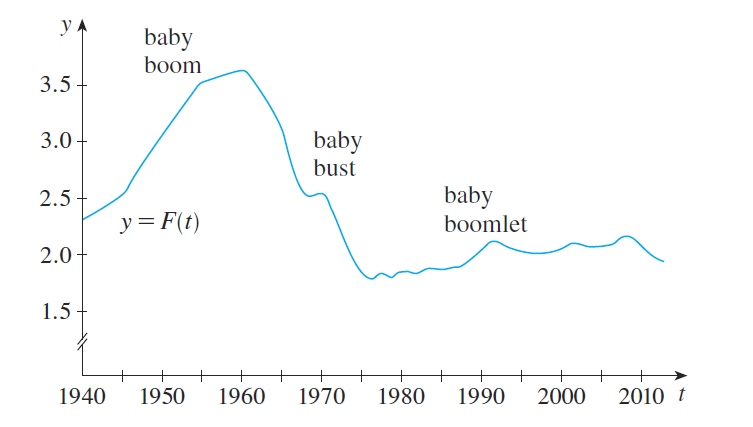#### Problem 8E

8. The total fertility rate at time $t$, denoted by $F(t)$, is an estimate of the average number of children born to each woman (assuming that current birth rates remain constant). The graph of the total fertility rate in the United States shows the fluctuations from 1940 to 2010 .

(a) Estimate the values of $F^{\prime}(1950), F^{\prime}(1965)$, and $F^{\prime}(1987) .$

(b) What are the meanings of these derivatives?

(c) Can you suggest reasons for the values of these derivatives?